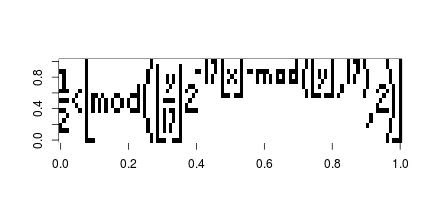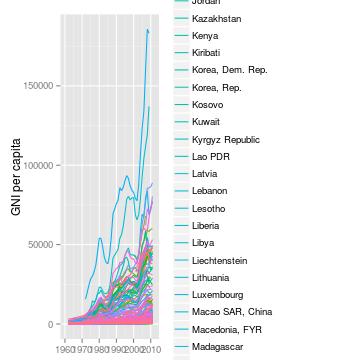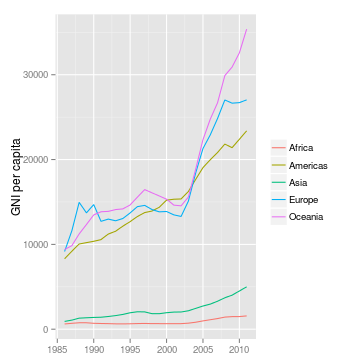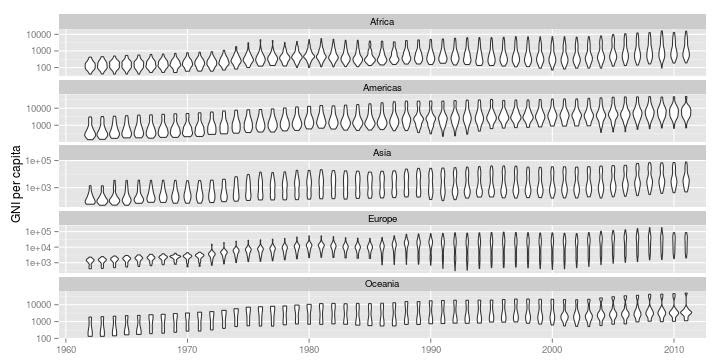## Wednesday, October 2, 2013

### First day of State of Food Insecurity (SOFI) 2013

The FAO flagship publication SOFI 2013 was release yesterday on the 1st of October, the publication is the most important report in monitoring the progress towards the 2015 Millenium Development Goal and ultimately eliminate hunger.

I was interest in how the people responded, so I scrapped some data from Twitter and previous work to carry out some basic analysis.

In total, there were 1,284 Tweets over the course of 24 hours. However, 86% were retweets which does not add much information for text mining and association analysis.

Nevertheless, lets have a look at what people are saying using some basic frequency and sentimental analysis. Clearly, hunger is the most mentioned words however there are words which are interesting and they have been colored according to theopinion lexicon of Hu and Liu. Negative feelings are red, positive feelings are blue while neutral words has remained grey.

The negative words like miss, bad and slow has pointed out that more efforts are required to achieve the MDG; furthermore people are suffer from chornic hunger. Nevertheless, the work of FAO has been recognised by good work and progress.

From the word cloud we can observe that it is impossible to use a single lexicon to generalize emotions. For example, fallen may usually used in a negative way but in this context it represents the positive movement of rate of undernourishment falling. A special lexicon may be required to examine the social feedback about SOFI.

Unfortunately I had problem obtaining the geocode from the API, otherwise it would be interesting to examine where these tweets originate.

Given that majority of the tweets are retweets of the official release message, little information can be obtained from these tweets. We hope a revision in a few weeks/months time will provide us with much more insight about the status of the publication.

The codes can be found at my Github repository .

## Tuesday, July 2, 2013

### Keys to win a basketball game

Offence sells tickets, defences wins games, rebounding wins championships - Pat Summitt

In fact, if we try to translate the game of basketball into mathematics (very naively) we have the following equation.

\begin{align} \text{Score advantage}_x &= \text{Possession}_x \times \text{Probability of Scoring}_x - \text{Possession}_y \times \text{Probability of Scoring}_y\\ &= [\text{out of bounds}_x + \text{rebound}_x] \times \text{Probability of Scoring}_x\\ &- [\text{out of bounds}_y + \text{rebound}_y] \times \text{Probability of Scoring}_y\\ &= [\text{out of bounds}_x + \text{rebound}_x] \times \text{Probability of Scoring}_x\\ &- [\text{out of bounds}_y + (\text{total rebound} - \text{rebound}_x)] \times \text{Probability of Scoring}_y \end{align}

From the equation we can see that a good scoring team has a high probability to score, while a good defensive team reduces the opponents ability and probability to score. Yet, we can see that the rebound appears on both end where a rebound for team x is one less rebound for team y. Which modifies the number of possession giving more chance to attack and a potentially larger score advantage!

Of course, rebounds can be offensive and defensive and they are a function of your and your opponents scoring ability. The events are inter-connected and should be modelled as a chained event. Maybe I'll do that when I recover from my hang-over.

## Sunday, March 24, 2013

### Tupper's self-referential formula

Can't remember where I first came across this equation but the Tupper's self referential equation, is a very interesting formula that when graphed in two dimension plane it reproduces the formula.

$\frac{1}{2} < \left\lfloor \bmod\left(\left\lfloor\frac{y}{17}\right\rfloor2^{-17\lfloor x\rfloor - \bmod(\lfloor y \rfloor, 17)}, 2\right)\right\rfloor$

I first thought this would be a quick 5 min exercise which turned into a 3 hour work, the obstacle was that the constant “k” used in the formula is an extremely big integer and can not be handled in R naturally.

After a little search Large integer in R and play around it seems that the gmp library seems to work well.

First you will need to install GMP (The GNU Multiple Precision Arithmetic Library) from http://gmplib.org/, then install the gmp library within R.

## Load the library after installing GMP
library(gmp)

## Define the constant k
k = as.bigz("960939379918958884971672962127852754715004339660129306651505519271702802395266424689642842174350718121267153782770623355993237280874144307891325963941337723487857735749823926629715517173716995165232890538221612403238855866184013235585136048828693337902491454229288667081096184496091705183454067827731551705405381627380967602565625016981482083418783163849115590225610003652351370343874461848378737238198224849863465033159410054974700593138339226497249461751545728366702369745461014655997933798537483143786841806593422227898388722980000748404719")

## The tupper's formula
tupper = function(x, y, k){
z1 = as.bigz(y + k)
z2 = as.bigq(z1/17)
z3 = 2^(-17 * x - as.bigz(z1%%17))
0.5 < floor(as.bigz(z2 * z3)%%2)
}

## The x and y axis
x = 0:105
y = 0:16

## Compute the matrix
a = matrix(0, nc = length(x), nr = length(y))
for(i in seq_along(x)){
a[ ,107 - i] = rev(tupper(x[i], y, k = k))
}


Here is the plot

image(t(a), col = c("white", "black"))## Wednesday, March 20, 2013

### Violin plots and regional income distribution

While preparing my slides for statistical graphics, a plot really caught my eye when I was playing around with the data.

I started off by plotting the time seriesof GNI per capita by country, and as expected it got quite messy and incomprehensible.

## Download and manipulate the data
library(FAOSTAT)
raw.lst = getWDItoSYB(indicator = c("NY.GNP.PCAP.CD", "SP.POP.TOTL"))
raw.df = raw.lst[["entity"]]
traw.df = translateCountryCode(raw.df, from = "ISO2_WB_CODE", to = "UN_CODE")
mraw.df = merge(traw.df, FAOregionProfile[, c("UN_CODE", "UNSD_MACRO_REG")])
final.df = mraw.df[!is.na(mraw.df$UNSD_MACRO_REG), ] ## Simple ugly time series plot ggplot(data = final.df, aes(x = Year, y = NY.GNP.PCAP.CD)) + geom_line(aes(col = Country)) + labs(x = NULL, y = "GNI per capita")So I decided to compute the weighted average by region to examine the regional trends. ## Compute regional aggregates based on UN M49 definition reg.df = aggRegion(aggVar = "NY.GNP.PCAP.CD", weightVar = "SP.POP.TOTL", data = traw.df, keepUnspecified = FALSE, aggMethod = "weighted.mean", relationDF = data.frame(UN_CODE = FAOregionProfile[, "UN_CODE"], REG_NAME = FAOregionProfile[, "UNSD_MACRO_REG"])) ## Plot regional aggregates ggplot(data = reg.df[!is.na(reg.df$NY.GNP.PCAP.CD), ],
aes(x = Year, y = NY.GNP.PCAP.CD)) +
geom_line(aes(col = REG_NAME)) +
labs(x = NULL, y = "GNI per capita", col = "")I can now see the trend clearly, but there are two problems with this approach. First, the variability within region is vast and thus the weighted average or any summary statistic such as quantile can be misleading and it does not tell me what is going on within the regions. Secondly, since a minimum of 65% of the country must be present in order to compute the aggregation, no statistics was available prior to 1985.

While I was carrying out regional comparisons with box-plot and violin plot I thought why not plot them accross time as well! So here is the final graph:

## Time series violin plot
ggplot(data = final.df,
aes(x = as.character(Year), y = NY.GNP.PCAP.CD)) +
geom_violin() + scale_y_log10() +
facet_wrap(~UNSD_MACRO_REG, ncol = 1, scales = "free_y") +
scale_x_discrete(breaks = as.character((seq(1960, 2010, by = 10))),
labels = as.character((seq(1960, 2010, by = 10)))) +
labs(x = NULL, y = "GNI per capita")Now I can compare the regions, but at the same time I can see the within region income distribution. It amazes me how the income distribution diverges in Europe and Oceania while America and Asia moves towards a bell shaped distribution. Growth in Africa appears to be slow, but there are several countries which are growing at a faster rate and pushing the tail of the distribution. Although some of the variability in the density may have resulted from independence of countries, nonetheless it is still infromative.

## Monday, February 11, 2013

### R package building automation

Title: R package building automation

Inspired by the post at http://giventhedata.blogspot.tw/2013/02/my-r-package-development-cheat-sheet.html. I have decided to publish my cheat script for package development as well.

Building package used to be a nightmare, filling in all those Rd files manually can cause some serious brain damage. Thanks to the roxygen2 team and knitr, building a package with nice documentation is merely running a script like what I have below. Just modify and change pkgName to the name of your package then your package should be ready in 5 minute.


## Build the package
## ---------------------------------------------------------------------

## Remove the folder if it exists
if(file.exists("./pkgName"))

## Build the package
package.skeleton("pkgName", code_files = paste("./Codes/",
dir("./Codes/", pattern = "\\.R$"), sep = ""), force = TRUE) ## Include the data dir.create("pkgName/data") file.copy(from = "./FAOcountryProfile.RData", to = "pkgName/data/", overwrite = TRUE) file.copy(from = "./FAOregionProfile.RData", to = "pkgName/data/", overwrite = TRUE) file.copy(from = "./FAOmetaTable.RData", to = "pkgName/data/", overwrite = TRUE) file.copy(from = "./DESCRIPTION", to = "pkgName/", overwrite = TRUE) ## Include Demo dir.create("pkgName/demo") file.copy(from = "./pkgNamedemo.R", to = "pkgName/demo/", overwrite = TRUE) cat("pkgNamedemo Demonstration for the pkgName package\n", file = "pkgName/demo/00Index") ## Use roxygen to build the documentation library(roxygen2) roxygenize("pkgName") ## Include vignette dir.create("./pkgName/inst/doc/") file.copy(from = "../Documentation/pkgName/pkgName.pdf", to = "./pkgName/inst/doc/", overwrite = TRUE) ## Create the vignette hack from (http://yihui.name/knitr/demo/vignette/) ## This is not required for R 3.0.0 cat("%\\VignetteIndexEntry{General Manual}\n\\documentclass{article}\n\\begin{document}\n\\end{document}", file = "./pkgName/inst/doc/pkgName.Rnw") ## Build and check the package system("R CMD INSTALL --build pkgName") system("R CMD build pkgName") system("Rcmd check pkgName") system("Rcmd check --as-cran pkgName")  PS: This is my first post using Rmd, the highlight for R is defintely much better than plain text. However, I think some twick is required for my blog theme. ## Tuesday, February 5, 2013 ### Relearn boxplot and label the outliers Despite the fact that box plot is used almost every where and taught at undergraduate statistic classes, I recently had to re-learn the box plot in order to know how to label the outliers. This stackoverflow post was where I found how the outliers and whiskers of the Tukey box plots are defined in R and ggplot2: In ggplot2, what do the end of the boxplot lines represent? and this post on how to label the outliers using base graphics. How to label all the outliers in a boxplot Since the use of ggplot2 is required for this task, I have written some basic hack code to label the outliers for ggplot2. Here are the codes:  ## Install the FAOSTAT package to obtain the data if(!is.element("FAOSTAT", .packages())) install.packages("FAOSTAT") library(FAOSTAT) ## Download data on Cassava production cp.lst = getFAOtoSYB(name = "cassava_production", domainCode = "QC", itemCode = 125, elementCode = 5510) ## Use the country level data, and take only data for 2011 and remove the NA's cp.df = cp.lst$entity[!is.na(cp.lst$entity$cassava_production) &
cp.lst$entity$Year == 2011, ]

## Merge with the country profile to obtain the country names for labelling
ccp.df = merge(cp.df, FAOcountryProfile[, c("FAOST_CODE", "ABBR_FAO_NAME")],
all.x = TRUE)

## Merge with the regional pofile to obtain the UNSD M49 macro region
## composition for multiple boxplot.
rcp.df = merge(ccp.df, FAOregionProfile[, c("FAOST_CODE", "UNSD_MACRO_REG")],
all.x = TRUE)

## Compute the quantile
qrcp.df = ddply(.data = rcp.df, .variables = .(UNSD_MACRO_REG), transform,
lQntl = quantile(cassava_production, probs = 0.25, na.rm = TRUE),
uQntl = quantile(cassava_production, probs = 0.75, na.rm = TRUE))

## Compute the lower and upper bound which defines the outlier
brcp.df = ddply(.data = qrcp.df, .variables = .(UNSD_MACRO_REG), transform,
lBound = lQntl - 1.5 * (uQntl - lQntl),
uBound = uQntl + 1.5 * (uQntl - lQntl))

## Remove the country names if it is within the bounds
with(brcp.df, {
brcp.df[cassava_production <= uBound &
cassava_production >= lBound, "ABBR_FAO_NAME"] <<- NA
})

## Plot the data
set.seed(587)
ggplot(data = brcp.df, aes(x = UNSD_MACRO_REG, y = cassava_production)) +
geom_boxplot(outlier.colour = NA) +
geom_text(aes(label = ABBR_FAO_NAME), size = 2,
position = position_jitter(width = 0.1)) +
labs(x = NULL, y = NULL, title = "Production of Cassava by region")


Here is the final product, to avoid over-plotting of texts I have used position_jitter. Which is not an elegant solution but I just can not find any algorithm that works well in general.

## Sunday, February 3, 2013

### A package for agricultural statistic: FAOSTAT

After 8 years of using R, today I finally become a contributor to the community and released my first package, FAOSTAT.

The package is designed to provide user with direct access to the FAOSTAT data base via R and to support the open data and methodology philosophy used in the Statistical Yearbook of the Food and Agricultural Organization.

The package can be downloaded and installed the same way as other package in a single line:
install.packages("FAOSTAT")

Or the latest development version from github:
 library(devtools)
install_github("FAOSTAT","mkao006")


A draft documentation can be found at:

vignette("FAOSTAT", package = "FAOSTAT")


I hope the package will save time for people working with agricultural statistics and more generally official statistics and to promote the use for research. All feedbacks and criticisms are welcome.

### Maize trade Part II: Comparison and analysis

Following my last post about the maize network, although interesting but is not very informative. What we are going to do today is to contrast the maize network with the wine trade network.

The choice why we have chose wine will become clear after the network and the analysis. Lets first have a look at the trade network of wine, again only the top 80% of the trade has been shown.

First, we can see that the wine network is much more inter-connected than the maize network. Secondly, the amount of reciprocal trading is much higher. Third, there does not appear to have a single trading of the size of the magnitude such as the export of maize from United state to Japan and Korea

The advantage of the network diagram not only reveals the connection, but the structure of the network, hence the nature of the market. It is clear that the market for maize and wine is different, but how, and why, is what we are interested.

Usage/consumption:
First of all, the usage of the two commodity are on the opposite end of the spectrum. Maize is a staple crop, necessary in many regional cuisine also used for livestock feed and bio-fuel. On the other hand, wine is consumed at a lesser extent and is considered as a luxury or enjoyment (although considered a necessity in Italy and several other countries).

Cost:
The usage of the commodity lead to the difference in cost and elasticity. Staples and biofuel need to be cheap to be viable and thus cost is an important consideration. In contrast, the preference and quality of wine plays a much bigger role than the actual price tag. This is where the trade agreement plays a significant role in promoting the trade of maize between the United States, Japan and Korea but a smaller element for wine trading.

Finally, the variety and the value added of the commodity characterizes the trade. Japan can import maize from any country in the world to use for consumption, feed or to produce bio-fuel without any considerable change or impact because the value add of maize is small; simply put, the product can be easily substituted. On the other hand, wine is dominated by several producers such as France, Italy and a handful of new world wine producers. Which is not easily substituted given the preferences. This is the contributing reason to the high number of trading partners, interconnected clusters and reciprocal trading; since a single trading partner can not satisfy the preferences of the consumer and even the French would like to have Italian wine occasionally.

This example illustrate how a simple network diagram can reveal a lot of interesting insight compare to analyzing just the quantity and value of trade, it also supports my view on how important exploratory analysis is.


stringsAsFactors = FALSE)

wineEx.df = subset(wine.df,subset = type == "Export",
c("reporting_country", "partner_country", "Wine"))

## Sort the data and take only the top 80% of the trade
wineEx.df = arrange(wineEx.df, desc(Wine))
wineEx.df$sWine = scale(wineEx.df$Wine, center = FALSE)
wineEx.df$cs = cumsum(wineEx.df$Wine)
wineEx.df[wineEx.df[, 1] == "China, Hong Kong SAR ", 1] = "China, Hong Kong SAR"
wineFinal.df = subset(wineEx.df, cs < tail(wineEx.df$cs, 1) * 0.8) ## Set edge and arrow size wineFinal.df$edgeSize = with(wineFinal.df, Wine/sum(Wine))
wineFinal.df$arrowSize = ifelse(wineFinal.df$edgeSize * 30 < 0.5 , 0.5,
wineFinal.df$edgeSize * 15) ## Create the network and plot wine.net = network(wineFinal.df[, 1:2]) plot(wine.net, displaylabels = TRUE, label.col = "steelblue", edge.lwd = c(wineFinal.df$edgeSize) * 100,